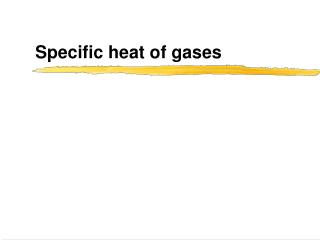# Specific heat of gases - PowerPoint PPT PresentationDownload PresentationSpecific heat of gases

Specific heat of gases
Download Presentation## Specific heat of gases

- - - - - - - - - - - - - - - - - - - - - - - - - - - E N D - - - - - - - - - - - - - - - - - - - - - - - - - - -
##### Presentation Transcript

1. Specific heat of gases

2. Specific heat of monatomic gas I • Add heat keeping the volume constant. Energy can only go into kinetic energy of atoms: • Conclusion:

3. Specific heat of a monatomic gas II • Add heat keeping the pressure constant. Energy can go into kinetic energy of atoms, or into work when the gas expands: • Some mathematical gymnastics:

4. Specific heat of a monatomic gas III • Substitute: • Conclusion: • Valid for all ideal gases

5. Equipartition of energy • According to classical mechanics, molecules in thermal equilibrium have an average energy of associated with each independent degree of freedom of their motion provided that the expression for energy is quadratic. • monatomic gas: x, y, z motion: since the terms are quadratic

6. Degrees of freedom for molecules • We take m to be the mass of the molecule, then as for monatomic gases • However, there is now internal energy due to rotation and vibration:

7. Diatomic molecule: rotation • Think of molecule as dumbbell: • Centre-of-mass (CM) moves with K.E. • Rotation about CM with K.E. • All quadratic: f = 6

8. Atoms attract each other via Lennard-Jones potential Atoms stay close to equilibrium Can be approximated by a harmonic oscillator potential Diatomic molecule: vibration

9. Harmonic oscillator • Average P.E. = average K.E. • P.E. is quadratic so associated with it • K.E. is the same so also • Total: kT or f = 2.

10. Diatomic molecules: conclusion? • According to classical physics there should be 8 degrees of freedom for each diatomic molecule… • From Cp and CV measurements for H2: • below 70 K: f = 3 • at room temperature: f = 5 • above 5000 K: f = 7

11. Question • If e.g. vibration of H2 does not contribute to the specific heat, then that is because a) The vibration is independent of temperature b) The vibration doesn’t change in collisions c) The vibration is like a very stiff spring

12. Quantum mechanical effects • Translation: 3 degrees • Rotation: 2 degrees • Vibration: • at room temperature often also “frozen out” • at high temperatures K.E. + P.E. = 2 degrees Rotation about these axes imparts energy Rotation about this axis is “frozen out”

13. Quantum mechanics: rotational and vibrational energies not continuous but quantised Rotation: spacing ~1/I small steps, near-continuous about two axes big steps about axis through atoms If step >> kT : not excited, no rotation Even at T=104 K no rotation about third axis Rotational states

14. Vibration: harmonic oscillator Spacing ~1/m step >> kT for light molecules (O2, N2) but excitations possible for heavy molecules such as I2 Potential energy: on average equal to kinetic energy vibration contributes kT when it does Vibrational states

15. Solids • Model for solid: • Elemental (“monatomic”) solids have K.E. and P.E. to give C = 3R (Rule of Dulong and Petit)

16. Freezing out - again • At lower temperatures, some vibrational levels cannot be reached with kT energy. At very low temperatures C T3. • For metals the electrons (which can move freely through the metal) contribute and C= aT + bT3 (Einstein-Debye model)

17. Dulong & Petit: elemental solids C  25 J mol-1 K-1 Specific molar heat

18. Question 2 • A room measures 4.002.402.40 m3. Assume the “air molecules” all have a velocity of 360 ms-1 and a mass of 510-26 kg. The density of air is about 1 kg m-3. Calculate the pressure in this room.

19. Isothermal expansion of an ideal gas • Isothermal expansion: pressure drops as volume increases since pV = nRT = constant • The internal energy only depends on the temperature so it doesn’t change • Two equations hold at the same time: pV = nRT and T2 = T1

20. Question • An identical volume of the same gas expands adiabatically to the same volume. The pressure drops a) more than in the isothermal process b) by the same amount c) less than in the isothermal process

21. Adiabatic expansion of an ideal gas I • Q = 0 so U + W = 0 • Remember: U = nCV T • Use ideal gas law: • Substitute:

22. Adiabatic expansion of an ideal gas II • Divide by nCV T: • Prepare for integration: • Define so that

23. Adiabatic expansion of an ideal gas III • Integrate: • Play around with it: • Use

24. Question • Consider isothermal and adiabatic expansion of a Van der Waals gas. Do T2 = T1 and pVg = C hold for this gas? a) yes; yes b) yes; no c) no; yes d) no; no

25. Question • A gas is compressed adiabatically. The temperature a) rises because work is done on the gas b) rises depending on how much heat is added c) drops because work is done by the gas d) drops depending on how much heat is added

26. PS225 – Thermal Physics topics • The atomic hypothesis • Heat and heat transfer • Kinetic theory • The Boltzmann factor • The First Law of Thermodynamics • Specific Heat • Entropy • Heat engines • Phase transitions